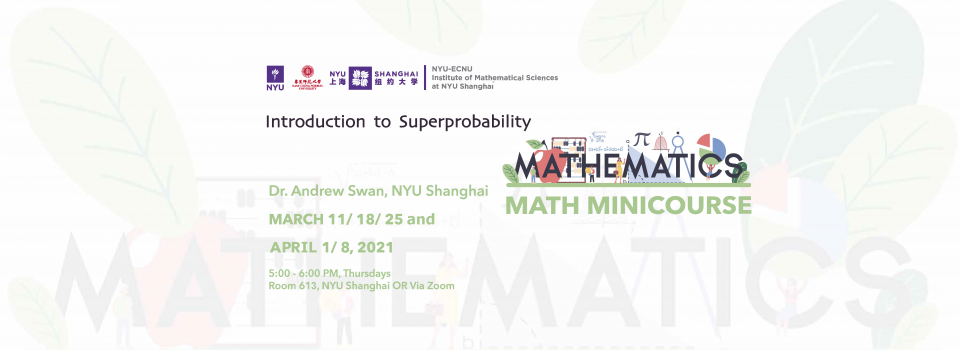# Introduction to Superprobability (Course I)Topic:
Introduction to Superprobability (Course I)
Date & Time:
Thursday, March 11, 2021 - 17:00 to 18:00
Speaker:
Andrew Swan, NYU Shanghai
Location:
Via Zoom (Member of NYU Shanghai community can join from Room 613 at Pudong campus)

- RSVP Here -

Abstract:
In this mini-course, we will give an introduction to the mathematics of supersymmetry and how it can be used to tackle problems in probability theory. We will begin by introducing the language and world of supermathematics, with the goal of developing the complete suite of superanalytic tools necessary for the study of supersymmetric spin systems. Highlights here include the beautiful localisation lemma and the meaning of integration on spaces with negative dimension. Subsequently, we show how these supersymmetric spin systems can then be used to study problems arising in ordinary probability theory. The focus will be on local times of non-Markovian random walks, with some further applications to random spanning trees and forests on graphs.

Biography:
Prior to joining NYU Shanghai as a Faculty Fellow in 2020, Andrew completed his Ph.D. at the University of Cambridge. His current research lies at the crossroads of probability theory and mathematical physics, with a focus on supersymmetric statistical mechanics and non-Markovian random walks. Outside of this, he has a broader interest in random graphs, random matrix theory, and critical phenomena.

- Download the Flyer -

Math Mini-Course by the NYU-ECNU Institute of Mathematical Sciences at NYU Shanghai